Courses

# Quality Characteristics of Water Civil Engineering (CE) Notes | EduRev

## Civil Engineering (CE) : Quality Characteristics of Water Civil Engineering (CE) Notes | EduRev

The document Quality Characteristics of Water Civil Engineering (CE) Notes | EduRev is a part of the Civil Engineering (CE) Course Topic wise GATE Past Year Papers for Civil Engineering.
All you need of Civil Engineering (CE) at this link: Civil Engineering (CE)

Q. 1  Analysis of a water sample revealed that the sample contains the following species.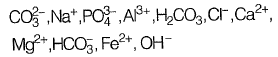Concentrations of which of the species will be required to compute alkalinity?   [2019 : 1 Mark, Set-II]
(a)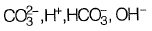(b)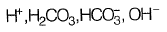(c)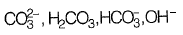(d)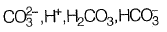Ans:  (a)
Alkalinity is defined as ability of water to neutralize the acid or hydronium ion
Alkalinity (AT) of water =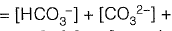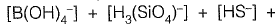[organic anions]+ [OH-]+ [H+]
From given options of ions in problem answer is (a).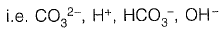Q. 2  A water sample analysis data is given below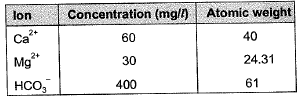The carbonate hardness (expressed as mg/L of CaC03, up to one decimal place) for the water sample is ______  [2018 : 2 Marks, Set-I]

Ans: 273.4 mg/l

Carbonate hardness = min. (Total hardness, alkalinity)

Total hardness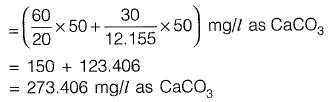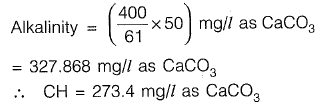Q. 3 As per IS 10500:2012, for drinking water in the absence of alternate source of water, the permissible limits for chloride and sulphate, in mg/L, respectively are   [2018 : 1 Mark, Set-II]
(a) 250 and 200
(b) 1000 and 400
(c) 200 and 250
(d) 500 and 1000

Ans: (b)
As per IS-10500 : 2012, Table 2
Permissible limit in absence of alternate source.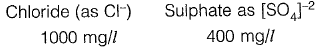Q. 4 The analysis of a water sample produces the following results: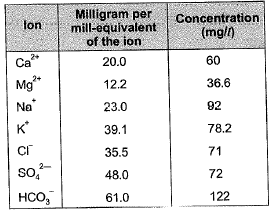The total hardness (in mg/l as CaC03) of the water sample is _______.    [2017 : 2 Marks, Set-I]
Ans: 300mg/l

Milligrah per milli-equivalent of the ion
= equivalent weight
∴ Milliequivalent of Ca2+ = 60/20 = 3
and, milliequivalent of Mg+2 36.6/12.2 = 3

Total hardness (due to Mg2+ and Ca2+)
= Milliequivalent of Ca2+ and Mg2+
= 3 + 3 = 6 milliequivalent/l
= (6 x 50) mg/l as CaC03
= 300 mg/l as CaC03

Q. 5 Effluent from an industry ‘A’ has a pH of 4.2. The effluent from another industry 'B' has double the hydroxyl (OH-) ion concentration than the effluent from industry ‘A'. pH of effluent from the industry ‘B’ will be _________     [2016 : 2 Marks, Set-I]
Ans: 4.5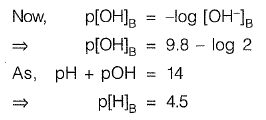Q. 6  A sample of water has been analyzed for common ions and results are presented in the form of a bar diagram as shown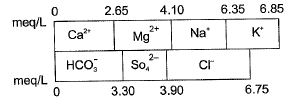The non-carbonate hardness (expressed in mg/l as CaCO3) of the sample is      [2016 : 2 Marks, Set-Il]
(a) 40
(b) 165
(c) 195
(d) 205
Ans: (a)

Hardness is due to multivalent metallic cations,
i.e. Ca2+ and Mg2+

Total hardness (mg/l as CaC03)
= (Total meq/l) x (eq. weight of CaC03 in mg)
= (4.1) x 50 mg/l as CaC03
= 205 mg/l as CaC03

Alkalinity is due to the presence of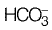in this case
Alkalinity (mg/l as CaC03)
= 3.3 x 50 mg/l as CaC03
= 165 mg/l as CaC03 Now, non-carbonate hardness
= Total Hardness in excess of alkalinity
= 205 - 165

= 40 mg/l as CaC03

Q. 7  A groundwater sample was found to contain 500 mg/L total dissolved solids (TDS). TDS (in %) present in the sample i s _______ . [2015 : 1 Mark, Set-II]
Ans: 0.05%
1 kg = 1 litre
TDS (in %) in the sample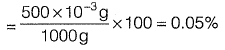Q. 8  For a sample of water with the ionic composition shown in the figure below, the carbonate and noncarbonate hardness concentrations (in mg/I as CaCO3), respectively are:       [2014 : 2 Marks, Set-I]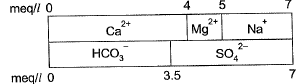(a) 200 and 50
(b) 175 and 75
(c) 75 and 175
(d) 50 and 200

Ans: (b)

Total hardness
= 5 meq/l (due to Ca2+ and Mg2+)
= (5 x 50) mg/l as CaC03
= 250 mg/l as CaC03

Alkalinity (due to HCO3-)

= 3.5 Meq/l
= (3.5x50) mg/l as CaC03
= 175 mg/l as CaC03

Carbonate hardness
= Min. (tota hardness, Alkalinity)
= Minimum (250,175)
= 175 mg/l as CaC03
Non-carbonate hardness
= Total hardness - Carbonate hardness
= 250-175

= 75 mg/l as CaC03

Q. 9  Some of the nontoxic metals normally found in natural water are
(a) arsenic, lead and mercury   [2014 : 1 Mark, Set-I]
(b) calcium, sodium and silver
(d) iron, manganese and magnesium
Ans: (d)

Although manganese is toxic, but only in very high concentration. And it is also a necessary consituent is water.

Hence, according to the options available, best possible answer is (d)

Q. 10  Match the given water properties in Group-I to the given titrants shown in Group-ll.    [2013 : 1 Mark]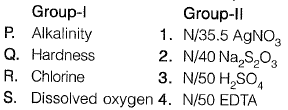(a) P-1, Q-2, R-3, S-4
(b) P-2, Q-1, R-4, S-3
(c) P-3, Q-4, R-1, S-2
(d) P-4, Q-3, R-2, S-1

Ans: (c)

Q. 11 A water sample has a pH of 9.25. The concentration of hydroxyl ions in the water sample is    [2012 : 2 Marks]
(a) 10-9.25moles/L
(b) 10-4.75 moles/L
(c) 0.302 mg/L
(d) 3.020 mg/L
Ans:
(c)
pH = 9.25
∵ pH + pOH = 14
⇒ pOH = 1 4 - 9 . 2 5 = 4.75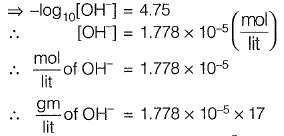= 30.226 x 10-5
= 30.226 x 10-6 gm/lit
∴ [OH-] = 302.6 x 10-3 mg/lit
= 0.302 mg/lit

Q. 12 Anaerobically treated effluent has MPN of total coliform as 106/100 mL. After chlorination, the MPN value declines to 102/100 mL. The percent removal (%R) and log removal (log R) of total coliform MPN is    [2011 : 1 Mark]
(a) %R = 99.90; log R = 4
(b) %R = 99.90; log R = 2
(c) % R = 99.99; log R = 4
(d) % R = 99.99; log R = 2

Ans: (c)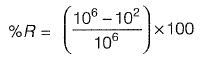= 0.9999 x 100 = 99.99%
log R = log 106 - log 102
= 6 - 2 = 4

Q. 13 Ion concentrations obtained for a groundwater sample (having pH = 8.1) are given below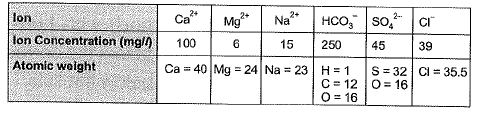Carbonate hardness (mg/L as CaC03) present in the above water sample is
(a) 205
(b) 250
(c) 275
(d) 289    [2010 : 2 Marks]
Ans: (a)

Total alkalinity in water consists of alkalinity caused by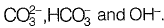A little negative alkalinity is also caused by H+.
∴ Total Alkalinity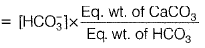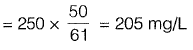Carbonate hardness is equal to the total hardness or alkalinity, whichever is less. The carbonate hardness of the sample will be 205 mg/L Non-carbonate hardness
= Total hardness - carbonate hardness
= 275 - 205 = 70 mg/L

Q. 14 Ion concentrations obtained for a groundwater sample (having pH = 8.1) are given belowTotal hardness (mg/L as CaC03) present in the above water sample is

(a) 205
(b) 250
(c) 275
(d) 308 [2010 : 2 Marks]
Ans: (c)

Total hardness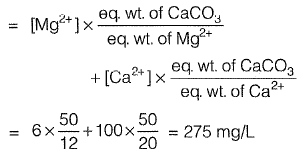Offer running on EduRev: Apply code STAYHOME200 to get INR 200 off on our premium plan EduRev Infinity!

67 docs

,

,

,

,

,

,

,

,

,

,

,

,

,

,

,

,

,

,

,

,

,

;